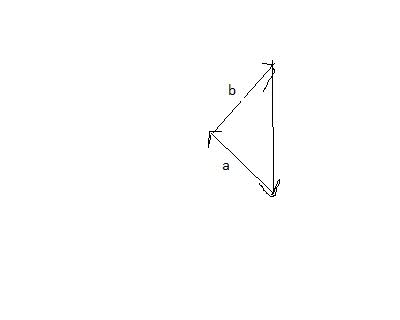# Finding Vector with Known Vector and Resultant Vector

deathcabislov

## Homework Statement

There are two forces. One has a magnitude of 8 N and acts at an angle of 30 degrees on the resultant vector. The resultant vector is 15 N.

## Homework Equations

equations for components (|a|cosx for horizontal and |a|sinx for vertical)
cos law and sin law
basic trig

## The Attempt at a Solution

First, I drew a diagram. Because the direction of the resultant is not stated, I positioned it going south. I then drew the known vector 30 degrees from the tail of the resultant. So it's tip acted 30 degrees on the tail of the resultant, as stated in the problem. I labelled that as b. Then I drew in the unknown vector, which I labelled as a. It makes an unknown angle with the resultant and has an unknown magnitude.
I tried using the cos law/sin law way of solving this, but all I could figure out is that vector a makes an angle of 60+x degrees with vector b.
I also tried solving with components. I calculated 4N and 7N for the hor. and vert. components of b, respectively and 0N and 15N for the resultant. But after this I am completely lost!

I would really appreciate some feedback just on how I have my diagram set up and maybe some tips on where to go from here, if I have anything correct! Thank you!

Homework Helper
welcome to pf!

hi deathcabislov! welcome to pf!There are two forces. One has a magnitude of 8 N and acts at an angle of 30 degrees on the resultant vector. The resultant vector is 15 N.

First, I drew a diagram. Because the direction of the resultant is not stated, I positioned it going south. I then drew the known vector 30 degrees from the tail of the resultant. So it's tip acted 30 degrees on the tail of the resultant, as stated in the problem. I labelled that as b. Then I drew in the unknown vector, which I labelled as a. …

If there are two forces, (b = 8 N, and a unknown) and their resultant is 15N, then shouldn't b go on the end of a, not on the end of the 15 N ?deathcabislov

Thank you! :)

If there are two forces, (b = 8 N, and a unknown) and their resultant is 15N, then shouldn't b go on the end of a, not on the end of the 15 N ?Yes, that is what I meant. Here is a very messy depiction of what I have:I know this is an easy problem but I'm so confused!

Homework Helper
hi deathcabislov!I also tried solving with components. I calculated 4N and 7N for the hor. and vert. components of b, respectively and 0N and 15N for the resultant.

well, that should work (of course, it isn't exactly 7 N) …

show us what you did after thatdeathcabislov
hi deathcabislov!well, that should work (of course, it isn't exactly 7 N) …

show us what you did after thatHaha. No. It's 6.92820323 N. But for this question, all I need to use is 7 N.

Well, I tried to use the Pythagorean Theorem, because the square of the resultant equals the square of the sum of the x-components plus the square of the sum of the y-components.
So:
(15 N)2=(ax+4N)2+(ay+7N)2

Homework Helper
(15 N)2=(ax+4N)2+(ay+7N)2

noooo, that's nonsensethat would be a formula for a right-angled triangle with 15 on the hypotenuse, wouldn't it?

you need to find ax and ay by subtraction(in vectors, it's a + b = (0,15))

deathcabislov
Ohhhhhhkay. And once I find those, I can find the magnitude of a. And then can I just use one of the component formulas to find the direction of a?

yup!